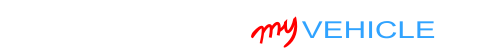## Interpreting Your Compression Test Results

On high mileage engines, it's not unusual to find that the cylinders have varying compression values.

If the variation in these values is low enough, you're not gonna notice any engine performance problems.

Thankfully, finding out if a low compression value is causing a misfire or a rough idle problem isn't hard.

The key to finding out if the lower compression value is causing a problem, is to find out if it's lower than 15% of the highest compression value you got.

You can do this (figuring out the 15%) in one of two ways: You can calculate this 15% difference with pen and paper or you can use my low compression calculator. You can find the low compression calculator here: Online Low Engine Compression Calculator (at: easyautodiagnostics.com).

If you want to manually calculate the 15% difference, here's what you'll need to do:

1. STEP 1: Multiply the highest compression value by 0.15 (this is the decimal value of 15%).
2. STEP 2: Round the result to the nearest one (for example: 25.6 would become 26).
3. STEP 3: Subtract the result (the number that was rounded) from the highest compression value.
4. ANSWER: The result of this subtraction is the lowest possible compression value any cylinder can have.

Now, let me give you a more specific example: Let's say that I got the following compression readings:

Cylinder Pressure
#1 165 PSI
#2   95 PSI
#3 155 PSI
#4 175 PSI

My next step is to do the following calculation:

1. STEP 1:  175 x 0.15 = 26.25.
2. STEP 2:  26.25 = 26 (rounded to nearest one).
3. STEP 3:  175 - 26 = 149.
4. ANSWER:  149 PSI. Any cylinder with this compression (or lower) value will misfire.

Since cylinder #2 is only producing 95 PSI, I can now conclude that it's 'dead' and causing a misfire.

To find out if the lowest compression value you got from your engine compression test is within a good range, you'll need to do the same calculation. Of course, you'll need to use the highest compression value you got and not the one in the example.

Once you've found the 'dead' cylinder, the next step is to find out what's causing the low compression value. For this step, go to: TEST 2: ‘Wet’ Engine Compression Test.

## TEST 2: Wet Compression TestIf in TEST 1 you found that one (or more) cylinders had low (or no) compression, the next step is to find out if that compression test result is due to worn cylinder head valves or worn piston rings.

We can do this by simply adding about 1 or 2 tablespoons of motor oil to the affected cylinders and retest them. The compression result that we're looking for (in the re-test after the oil has been added) is if the compression value goes up or not.

If the compression value goes up, then we can conclude that the low compression (in that cylinder) is due to worn piston rings. If the compression value does not go up, then we can conclude the problem is in the cylinder head valves.

At the bottom, I'll show you how to interpret your test results.

OK, this is what you'll need to do:

1. 1

Add a tablespoon of engine oil in the cylinder you need to retest. I suggest using a small and long funnel so that the oil will reach the inside of the cylinder.

2. 2

Once you've added the oil, install the compression gauge. As before, just hand tighten it.

3. 3

Have your helper crank the engine till the needle stops climbing on the compression gauge.

4. 4

You'll see one of two results:

1.) The needle will climb higher than the previous compression number you recorded for this specific cylinder.

2.) The needle will not move at all or stay at the same number you recorded earlier.

5. 5

Repeat steps 1 thru' 4 on any other cylinder that needs to be tested.

Let's take a look at what your compression test results mean:

CASE 1: Your compression tester registered a higher value. This compression test result confirms that the low compression value registered in this cylinder in the dry test is due to worn piston rings.

CASE 2: Your compression tester DID NOT register a higher value (than the previous recorded value). This result tells you that the low compression value registered in this cylinder in the dry test is due to worn/damaged cylinder head valves.If this info saved the day, buy me a beer!#### Latest

Toyota Vehicles:

• Corolla 1.6L
• 1989, 1990, 1991, 1992, 1993, 1994, 1995, 1996, 1997

Geo Vehicles:

• Prizm 1.6L
• 1993, 1994, 1995, 1996, 1997